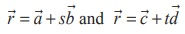Home | | Maths 12th Std | Condition for coplanarity of two lines

# Condition for coplanarity of two lines

Condition for coplanarity of two lines : (a) Condition in vector form (b) Condition in Cartesian form

Condition for coplanarity of two lines

(a) Condition in vector form

The  two  given non-parallel linesare  coplanar. So they lie in a single plane. Let A and C be the points whose position vectors areand. Then A and C lie on the plane. Sinceandare parallel to the plane,×is perpendicular to the plane. Sois perpendicular to×. That is,This is the required condition for coplanarity of two lines in vector form.

(b) Condition in Cartesian formThis is the required condition for coplanarity of two lines in Cartesian form.

Study Material, Lecturing Notes, Assignment, Reference, Wiki description explanation, brief detail
12th Mathematics : UNIT 6 : Applications of Vector Algebra : Condition for coplanarity of two lines |